## Local time-frequency analysis and short time Fourier transform

Time-frequency analysis plays a central role in signal analysis. Already long ago it has been recognized that a global Fourier transform of a long time signal is of little practical value to analyze the frequency spectrum of a signal. High frequency bursts for instance cannot be read off easily from. Transient signals, which are evolving in time in an unpredictable way (like a speech signal or an EEG signal) necessitate the notion of frequency analysis that is local in time.

In 1932, Wigner derived a distribution over the phase space in quantum mechanics [Wig32]. It is a well-known fact that the Wigner distribution of an-function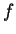is the Weyl symbol of the orthogonal projection operator onto[Fol89]. Some 15 years later, Ville, searching for an instantaneous spectrum'' - influenced by the work of Gabor - introduced the same transform in signal analysis [Vil48]. Unfortunately the non-linearity of the Wigner distribution causes many interference phenomena, which makes it less attractive for many practical purposes [Coh95].

A different approach to obtain a local time-frequency analysis (suggested by various scientists, among them Ville), is to cut the signal first into slices, followed by doing a Fourier analysis on these slices. But the functions obtained by this crude segmentation are not periodic, which will be reflected in large Fourier coefficients at high frequencies, since the Fourier transform will interpret this jump at the boundaries as a discontinuity or an abrupt variation of the signal. To avoid these artifacts, the concept of windowing has been introduced. Instead of localizingby means of a rectangle function, one uses a smooth window-function for the segmentation, which is close to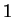near the origin and decays towards zero at the edges. Popular windows which have been proposed for this purpose are associated with the names Hamming, Hanning, Bartlett, or Kaiser. If the window is in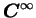(i.e. infinitely differentiable) one finds that for any-functionthe localized Fourier coefficients show at least polynomial decay in the frequency direction.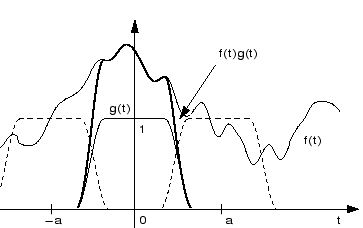The resulting local time-frequency analysis procedure is referred to as (continuous) short time Fourier transform or windowed Fourier transform. It is schematically represented in Figure 3. In mathematical notation, the short time Fourier transform (STFT) of an arbitrary function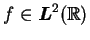with respect to a given (often compactly supported) windowis defined as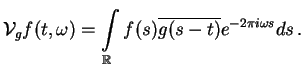The functioncan be recovered from its STFT via the inversion formulaIt is possible to derive the inversion formula (the integral is understood in the mean square sense) from the following formula, which itself can be seen as an immediate consequence of Moyal's formula. In particular it implies that for a normalized windowsatisfyingthe mapping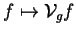is an isometric embedding frominto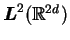The STFT and the spectrogramhave become standard tools in signal analysis. However the STFT has also its disadvantages, such as the limit in its time-frequency resolution capability, which is due to the uncertainty principle. Low frequencies can be hardly depicted with short windows, whereas short pulses can only poorly be localized in time with long windows, see also Figure 4 for an illustration of this fact. These limitations in the resolution were one of the reasons for the invention of wavelet theory.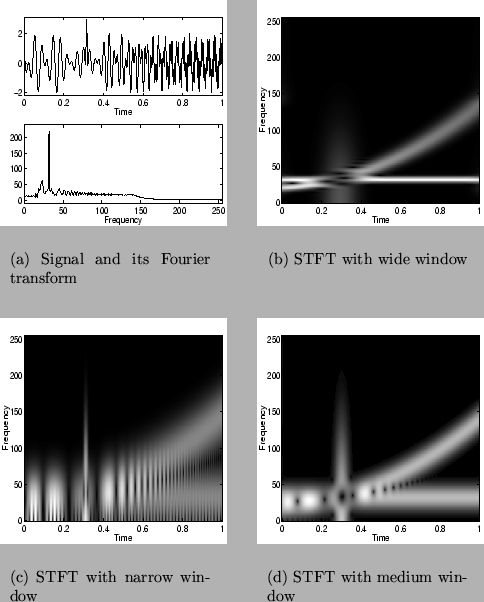Another disadvantage for many practical purposes is the high redundancy of the STFT. This fact suggests to ask, if we can reduce this redundancy by sampling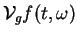. The natural discretization for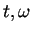is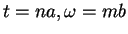where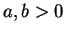are fixed, and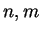range over, i.e., to sampleover a time-frequence lattice of the form.

Large values of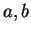give a coarse discretization, whereas small values oflead to a dense sampled STFT.

Using the operator notationand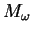for translation and modulation, respectively, we can express the STFT ofwith respect to a given windowasHence the sampled STFT of a functioncan also be interpreted as the set of inner products ofwith the members of the familywith discrete labels in the lattice. It is obvious that the members of this family are constructed in the same way as the representation functions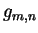in Gabor's series expansion. Thus the sampled STFT is also referred to as Gabor transform.

0 Thus the linear mappingwhere(4)

is also referred to as Gabor transform or Gabor analysis mapping, in analogy to the Gabor synthesis mapping defined in (0.6).

Two questions arise immediately with the discretization of the STFT

• Do the discrete STFT coefficients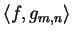completely characterize(i.e., doesfor allimply that)?
• A stronger formulation is: Can we reconstructin a numerically stable way from the?

Recall, that in connection with the Gabor expansion of a function we have asked

• Can any function inbe written as superposition of the elementary building blocks?
• How can we compute the expansion coefficients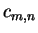in the series?

It turns out that the question of recoveringfrom the samples (at lattice points) of its STFT with respect to the windowis actually dual to the problem of finding coefficients for the Gabor expansion ofwith atom, using the same lattice to generate the time-frequency shifts of. Both problems can be successfully and mathematically rigorously attacked using the concept of frames and surprisingly for both questions the same dual'' Gabor atom has to be used.